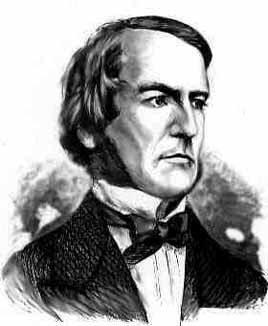# PIC10A Lecture 4

p44-61

## Boolean expressionIn 1854 he published An investigation into the Laws of Thought, on Which are founded the Mathematical Theories of Logic andProbabilities Boole approached logic in a new way reducing it to a simple algebra, incorporating logic into mathematics. He pointed out the analogy between algebraic symbols and those that represent logical forms. It began the algebra of logic called Boolean Algebra which now finds application in computer construction, switching circuits etc.
Examples
```bool a = true; bool b = 5; // number not equal to 0 means true bool c = 0; // false```
• Use `true` or `false`
• you can use integers to represent them
• `0` means `false`, any other number means `true`
• `cout` of a `bool` is `0` (if the `bool` is `false`) or `1` (if the `bool` is `true`).
C++ notation
English
Sample
```== ``` equal to
```x+7==2*y ```
```!= ``` not equal to
```ans != 'n' ```
```< ``` less than
```count < 1000 ```
```<= ``` less than or equal to
```time <= limit ```
```> ``` greater than
```time > limit ```
```>= ``` greater or equal than
```age >= 21 ```

### Logical operators

C++ notation
English
Sample
```&& ``` and
```(income > 20000) && (income < 30000) ```
```|| ``` or
```(grade=='A') || (grade=='B') ```
```! ``` not
`!(age < 21)`
The parentheses in the `&&` and `||`examples are not necessary

Question:
1) Which integers give an output `1`?
```int a = ????; cout << (a > 1 && a < 10 && a%2==1);```
2)
If `a = 3`, what is `!(a > 5 || a <7)?`

## if-else statements

Syntax
Meaning
Example
```if(condition)   one line statement;``` if condition is true, execute the statement.
```char gender; cin << gender; if(gender=='M' || gender=='m')     cout << "You are a male.\n"; ```
```if(condition) {     statement1;     statement2;     ......... }``` if condition is true, execute the statements. ```if(myScore > yourScore) {      ````cout << "I win!\n";````           myGameWin++;      }```

Remark about the braces { }
• You must use braces when there are more than one statements.
• When there is only one statement, the braces are optional.
• You can always put  braces (even for one line statement).
 ```/* Enter your score (within 0 to 100). The program will find out your grade. A: 90-100 B: 80-90 C: 65-80 D: 50-65 */ #include using namespace std; int main() {     double score;     cout << "Please enter your score: ";     cin >> score;     if(score>100 || score<0) { // score is within 0 to 100         cout << "Invalid score.\n";         cout << "Please check the score and run the program again.\n";     }     if(score>=90 && score<=100)         cout << "Your grade is A.\n";     if (score>= 80 && score<90)         cout << "Your grade is B.\n";     if (score >= 65 && score < 80)         cout << "Your grade is C.\n";     if(score>=50 && score<65)         cout << "Your grade is D.\n";     if(score>=0 && score<50)         cout << "You fail!\n";     return 0; }```

Syntax
Meaning
Example
```if(condition)     yes_statement; else     no_statement;```
If condition is true, execute yes_statement.
If condition is false, execute no_statement.
```char gender; cin << gender; if(gender=='M' || gender=='m')     cout << "You are a male.\n"; else     cout << "You are a female.\n"; ```
```if(condition) {    yes_statement1;    yes_statement2;    ...... }  else {     no_statement1;     no_statement2;    .... }```
If condition is true, execute yes_statements.
If condition is false, execute no_statemenst.
```if(myScore > yourScore) {      ````cout << "I win!\n";````           myGameWin++;      } else {      cout << "You win!\n";      yourGameWin++; } ```

Syntax
Meaning
```if(condition1) {    statement1;    ...... }  else if(condtion2){     statement2;    ..... }  ........ ............ else {    statement_for_other; }```

If condition1 is true, execute statement1.
If condition2 is true, execute statement2.
..........
if none of the above conditions is true, execute statment_for_other

Examples
 ```/* Enter your score (within 0 to 100). The program will find out your grade. A: 90-100 B: 80-90 C: 65-80 D: 50-65``` ```*/ #include using namespace std; int main() {     double score;     cout << "Please enter your score: ";     cin >> score;     if(score> 100 || score < 0) { // score is within 0 to 100         cout << "Invalid score.\n";         cout << "Please check the score and run the program again.\n";     } else if (score >= 90) {         cout << "Your grade is A.\n";     } else if (score >= 80) {         cout << "Your grade is B.\n";     } else if (score >= 65) {         cout << "Your grade is C.\n";     } else if(score >= 50) {         cout << "Your grade is D.\n";     } else {         cout << "You fail!\n";     }     return 0; }```